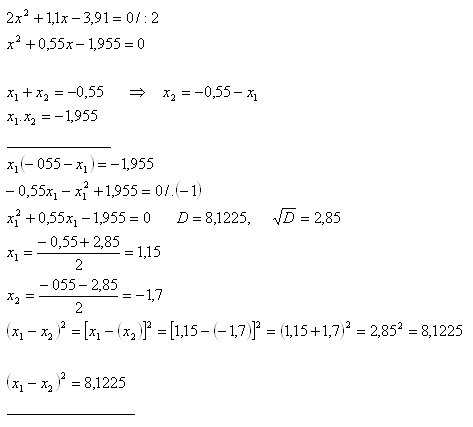sk|cz|

# Quadratic Equation - Roots Properties

1. Find out a quadratic equation in form  x2 +px +q = 0  so that p = -(x1+x2) and q = x1.x2 :

Solution: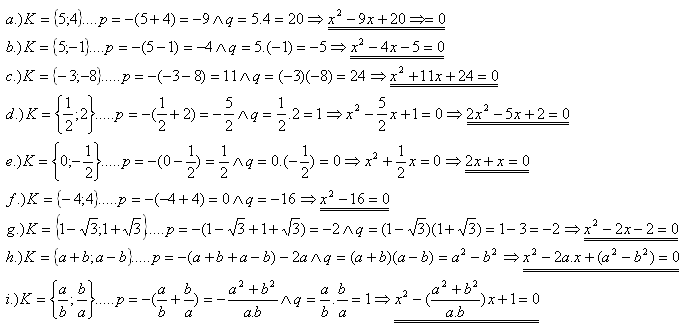2. For which „k“ one root of the equation x2-5x+k=0 equals x1=3?

Solution: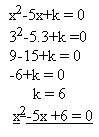3. For which „k“ one root of the equation kx2-15x+7=0 equals x1=7?

Solution: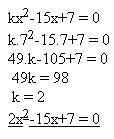4. For which „k“ one root of the equation x2+kx+20=0 equals x1=10?

Solution: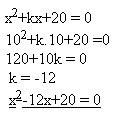5. For which „a“ the equations‘ roots fit the relation x2-3ax-4a2=0 and x1-x2=10?

Solution: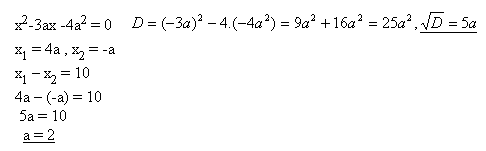6. For which „m“ real numbers does the equation x2+3x-2m2+m+3=0 have one zero root? What is the second root?

Solution: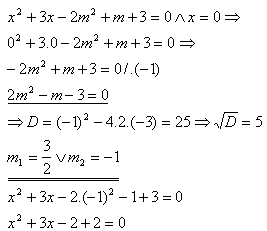7. For the equation x2-13x+36=0 find out an equation with roots y1,y2 which fit following relations: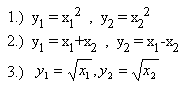Solution: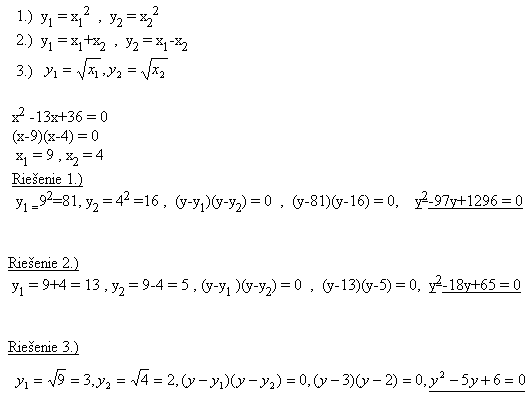8. For the equation x2 – 6x +8 = 0 find out an equation with roots that fit following relations: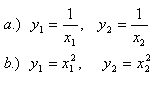Solution: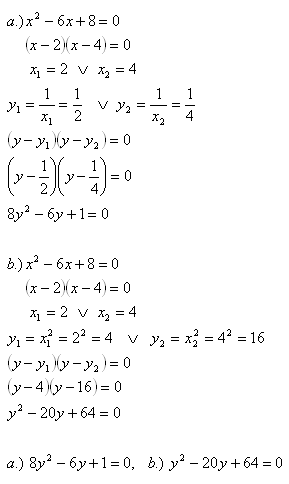9. The equation x2–x .cos α + cos 2α = 0 has roots x1 , x2. Express the relation: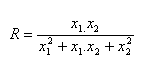Solution: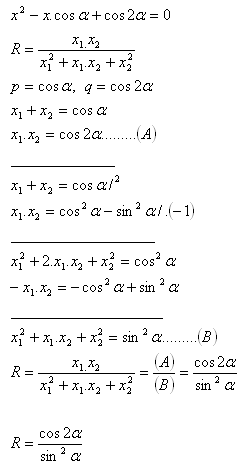10. Let x1 and x2 be the roots of the equation 2x2 + 1,1x - 3,91 = 0. Find out (x1 – x2)2, not solving the equation.

Solution: